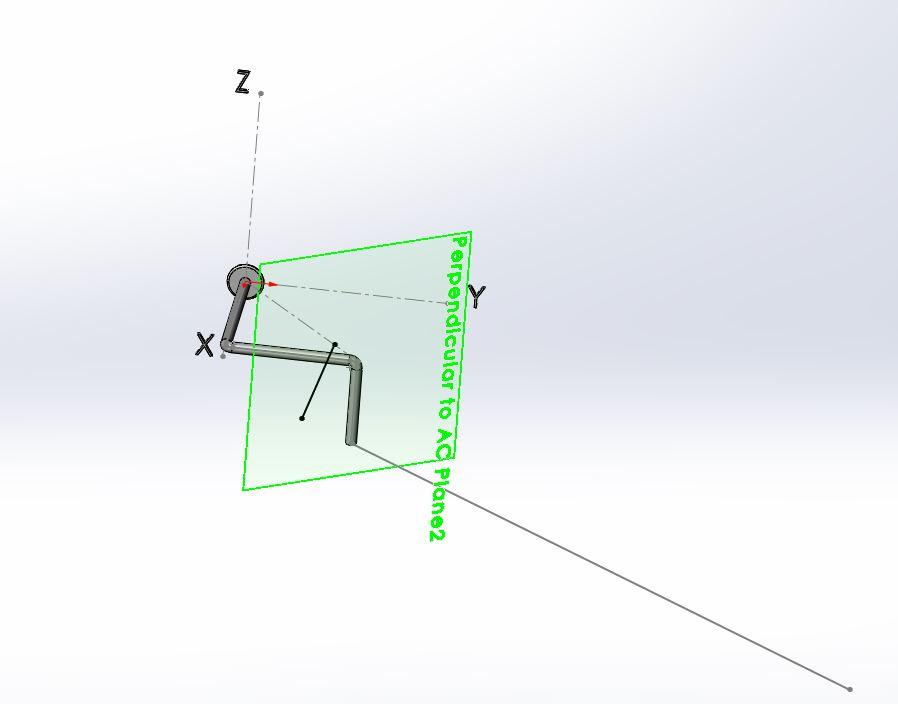# Cross & Dot Product Validated in 3D CAD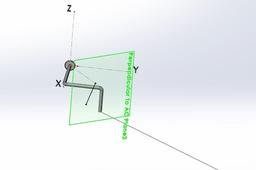Steps in Validating M = r X F and Maxis = U ●{​r X F}​ using 3D CAD (SolidWorks)
Using problem 3-43 and 3-44 from Hibbeler Statics & Mechanics of Materials 5th Edition.

1. ### Step 1: . Moment about Origin, A.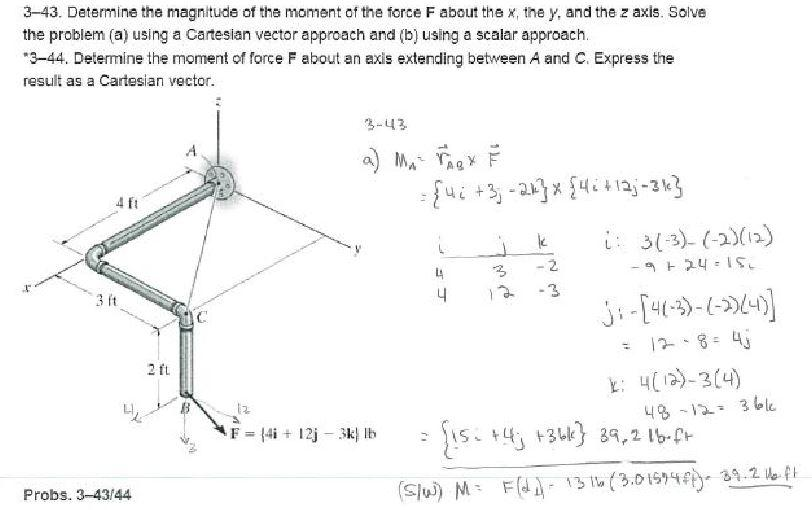MA = {rad X F}; which says the moment about A (the origin) is the position vector from A to D, cross product with the given force vector which is given as a Cartesian force vector.

= {4i + 3j – 2k} X {4i +12j – 3k}

I       j       k

4      3      -2

4      12    -3

I:      3(-3) - (-2)(12) = 15i

J:     -[4(3) - (-2)(4)] = 4j

k:    4(12) - 3(4)      = 36k

={15i + 4j +36k} 39.2 lb●ft

2. ### Step 2: Moment using 3D CAD

Verify the value using 3D CAD (SolidWorks)

a.  Down load the 3D model “Hibbeler3-43.prt” from my models in GrabCAD (to be added in the near future). Until then, you may want to create this model on your own after watching the video or referring to Hibbeler’s text.

b.  Measure the distance between the origin and the Force Vector, F= {4i + 12j -3k};

c.   Moment is the Force times the perpendicular distance which is 39.2 lb●ft; i.e. M = 13lb X 3.0157ft =39.2 lb●ft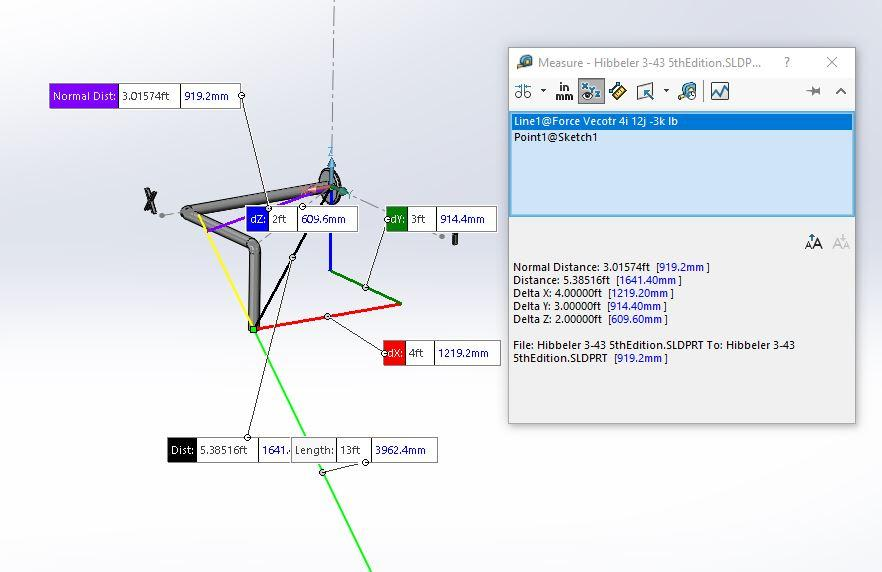3. ### Step 3: Moment about Axis, AC.

1.  Manual calculation for the Moment about the AC axis:

M = Uac ● {r X F};

a.  First determine the position vector from A to C:

rac = (4i + 3j}ft; 5ft;

b. So, the unit vector uac = {4/5i + 3/5j};

c.   The Dot product of this unit vector and the Moment Vector (already calculated in step 1 above), is the moment of the force about the axis AC. Mac = Uac ● {r X F}

d. {● {15i + 4j +36k} = [{ = 14.4}] lb●ft.

e.  Now, as a Cartesian Moment Vector,

Mac = {11.52i + 8.64j}lb●ft; 14.4 lb●ft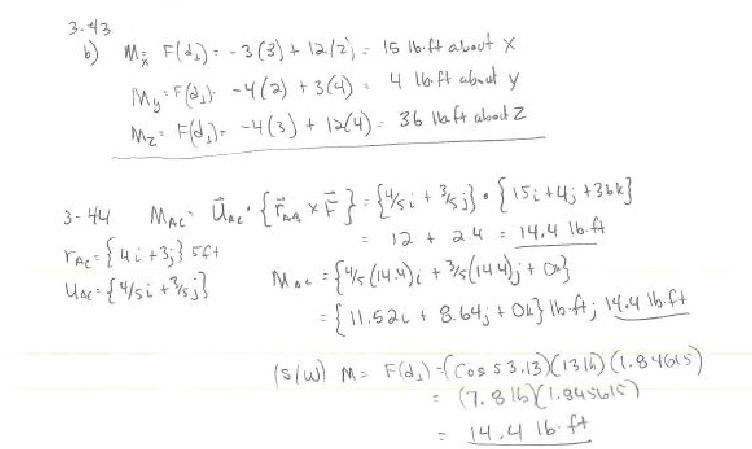4. ### Step 4: Validate Dot Product

1.  Now, let's verify this value using 3D CAD (SolidWorks).

a.  Using the model Hibbeler 3-43, create a plane normal to AC through the point at C; by Insert, Reference Plane, Select the Construction line between A and C; then Ctrl Select the point C. This new plane is perpendicular to AC.

b.  Measure the angle between this new plane and the Force Vector, {4i +12j – 3k} lb. which is about 53.13

c.   Determine the Cos of this angle which about .6

d.  Multiple .6 and the magnitude of the Force, .6 X 13 lbs=7.8 lbs

e.  Multiple this force and the perpendicular distance between the axisac and the force vector. One way to get this perpendicular distance is to insert a 3D sketch, then snap a line between AC and the Force vector. Select the new line, Ctrl Select the Force Vector and constrain them to be perpendicular. Then do the same between this new 3D sketch line and line AC. Select the new 3D sketch line and then Ctrl Select line AC and constrain them to be perpendicular to each other. You have now created a common perpendicular between a pair of skew lines.

So, the M = F X d = 7.8 lbs X 1.846 ft = 14.4 lb●ft which gives the same value as the Dot Product of unit vector of the axis and the Moment Vector determined by the cross product of r X F.